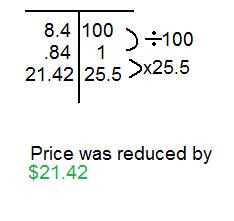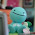## Tuesday, December 21, 2010

### Roemer's Scribe Post

3.) Use mental math to determine each of the following:

a.) 300% of 2000 = 6000

b.) 1 1/4% of 60 = 0.75

c.) 0.1% of 40 = 0.04

6.)
What is the percent of each number? Give your answer to the nearest hundredth.

a.) 5/8% of 520 = 3.25

b.) 75 2/5% of 200 = 150.8

c.) 250% of \$76.50 = \$191.25

8. The original price of a jacket was \$84.00. A store manager marked the price down by 25 1/2%. By how much was the price reduced?#### 1 comment:

1.Very understandable, and also very easy to read. Good choice of color and font size and also font. Great Job Roemer!